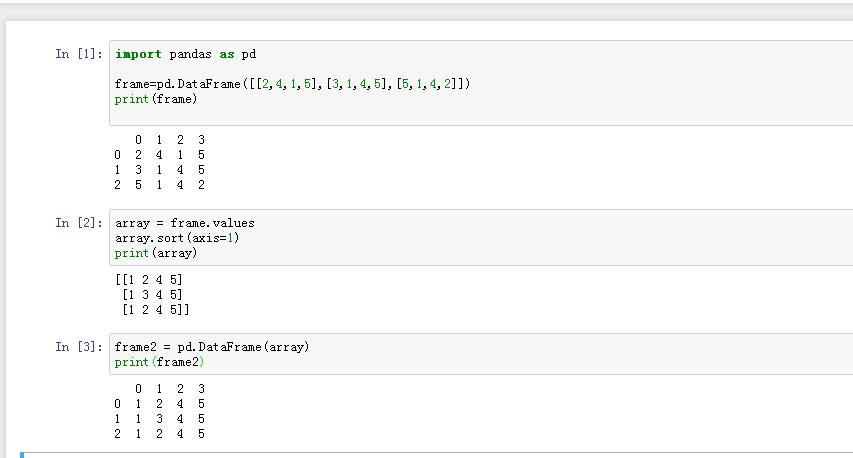pandas.dataframe 排序 5C

PS:不是指定行排序........

4个回答

LZ可以先将dataframe转换成array，对array进行按行排序，然后再把array转回dataframe
import pandas as pd

frame=pd.DataFrame([[2,4,1,5],[3,1,4,5],[5,1,4,2]])
array = frame.values
array.sort(axis=1)
frame2 = pd.DataFrame(array)df.sort_values(by=['列名1','列名2'])

1/默认的是什么。by=None吗
2/如果只希望排序的列改变，其他列不做改变，怎么做

6 8
3 2
4 6
1 5

1 8
3 2
4 6
6 5Python pandas 多重索引按不同需求排序

df = pd.DataFrame({'class':['A','A','A','B','B','B','C','C'], 'id':['a','b','c','a','b','c',a','b'], 'value':[1,2,3,4,5,6,7,8]}) df = df.set_index(['class','id']) 如何能对index排序，使class为升序，id为降序, 最后显示的结果类似于： class id value A c 3 A b 2 A a 1 B c 6 B b 5 B a 4 C b 8 C a 7

python中 dataframe使用permutation和take对 列 进行随机排序失败

pandas的dataframe的同一id的多行数据按其中一个特征将数据平铺该怎么简洁操作？

pandas：pivot_table计算占比

python分类时，利用groupby.sum()返回结果不是数字之和，而是数字重复罗列，这咋办？

<div class="post-text" itemprop="text"> <p>I have a list of dictionaries and want each item to be sorted by a specific property values.</p> <p>Take into consideration the array below,</p> <pre><code>[{'name':'Homer', 'age':39}, {'name':'Bart', 'age':10}] </code></pre> <p>When sorted by <code>name</code>, should become</p> <pre><code>[{'name':'Bart', 'age':10}, {'name':'Homer', 'age':39}] </code></pre> </div> <p>转载于:https://stackoverflow.com/questions/72899/how-do-i-sort-a-list-of-dictionaries-by-a-value-of-the-dictionary</p>

python 中pandas的value_counts是怎么算的？

pandas问题，分块读取，速度越来越慢慢

``` dataset = P.read_csv(r'E:\try\Stock_All.csv',chunksize = 4096,dtype ={'code':str}) for each in dataset: print('正在处理第 %d 块' % n) n += 1 unique = each['code'].unique() for each_code in unique: filedir = r'E:\try\S_A_Sep' +'\\'+ str(each_code) +'.csv' columns_need = ['date','open','high','close','low','volume','amount','code','id'] each[each['code'] == each_code].loc[:,columns_need].to_csv(filedir,mode ='a',header = columns_need) del each return None ``` 前1000块处理速度非常快，但到1500后面就非常慢 尝试用del each释放内存，没办法 有大神能解释下读取速度变慢的原因和解决方法吗

pandas清洗数据，假如日期数据中，标准格式是“xxxx-xx-xx”，要怎么把所有非标准的数据找出来呢？

python数据处理时遇到如下问题

import pandas as pd import numpy as np import matplotlib.pyplot as plt import csv import fileinput import time pd.options.display.max_columns=None start = time.time() data=pd.read_csv('C:\\Users\\丹心傲雪\\Desktop\\毕业论文冲鸭\\1001-CD\\1001-CD.txt') #The path of data file data.columns=['carid','orderid','time','longitude','latitude'] #添加列标签 orderid_list=np.array(data['orderid'].drop_duplicates()) #订单号列表 columns=['carid','orderid','starttime','endtime','longitude','latitude'] data_bak = pd.DataFrame(columns=columns) append_dic = {} data_end_time = [] for i in range(len(orderid_list)): order=data[data['orderid']==orderid_list[i]]#根据订单号筛选数据 order.sort_values("time",inplace=True) #对同一订单的时间进行排序 order=np.array(order) #将df转为array for j in range(len(order)): append_dic[columns[j]] = order[j] append_dic['endtime'] = order[-1] data_bak = data_bak.append([append_dic],ignore_index=True) data_bak.to_csv('data.csv',index=False) end = time.time() print("运行时间:%.2f秒"%(end-start)) ``` ```

python pandas group by以后 想根据某列count组中的最小值 去改变另外一列

![plt 图片](http://photo.weibo.com/5141700111/talbum/detail/photo_id/4341712832740349) 统计一个数据的异常点，用箱型图分析，异常点标注的是用圆圈，因为有几个值几乎重叠，圆圈看着太大了，看了官方文档也找不到在哪里改标注，不懂哪里修改成'+'。 ``` #-*- coding: utf-8 -*- import pandas as pd catering_sale = r'C:\Users\81284\OneDrive\python datamining\Python数据分析与挖掘实战\chapter3\demo\data\catering_sale.xls' #餐饮数据 data = pd.read_excel(catering_sale, index_col = u'日期') #读取数据，指定“日期”列为索引列 import matplotlib.pyplot as plt #导入图像库 plt.rcParams['font.sans-serif'] = ['SimHei'] #用来正常显示中文标签 plt.rcParams['axes.unicode_minus'] = False #用来正常显示负号 plt.figure() #建立图像 p = data.boxplot(return_type='dict') #画箱线图，直接使用DataFrame的方法 x = p['fliers'].get_xdata() # 'flies'即为异常值的标签 y = p['fliers'].get_ydata() y.sort() #从小到大排序，该方法直接改变原对象 #用annotate添加注释 #其中有些相近的点，注解会出现重叠，难以看清，需要一些技巧来控制。 #以下参数都是经过调试的，需要具体问题具体调试。 for i in range(len(x)): if i>0: plt.annotate(y[i], xy = (x[i],y[i]), xytext=(x[i]+0.05 -0.8/(y[i]-y[i-1]),y[i])) else: plt.annotate(y[i], xy = (x[i],y[i]), xytext=(x[i]+0.08,y[i])) plt.show() #展示箱线图 ```

MySQL数据库面试题（2020最新版）

6年开发经验女程序员，面试京东Java岗要求薪资28K

97年世界黑客编程大赛冠军作品（大小仅为16KB），惊艳世界的编程巨作

A站 AcFun弹幕视频网，简称“A站”，成立于2007年6月，取意于Anime Comic Fun，是中国大陆第一家弹幕视频网站。A站以视频为载体，逐步发展出基于原生内容二次创作的完整生态，拥有高质量互动弹幕，是中国弹幕文化的发源地；拥有大量超粘性的用户群体，产生输出了金坷垃、鬼畜全明星、我的滑板鞋、小苹果等大量网络流行文化，也是中国二次元文化的发源地。 B站 全称“哔哩哔哩(bilibili...

​能让德国人放弃现金支付​，也没谁了